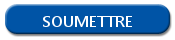# How to write a loop with R?

## Loops in R

In programming, loops are the elements that allow you to repeat the same action several times. These elements exist in R with two versions: the for loop and the while loop.

## Create a while loop in R

The while loop is a loop based on the respect of a condition. The condition is checked at the beginning of the loop.

As long as the condition is verified, the action on the loop is executed. Be careful, you must set an element on your loop to end it, otherwise you can create an infinite loop.

```while (condition == TRUE) {
print("My condition is true)
}```

## For loop in R

The for loop is an iterative loop:  a variable will have each value of a vector until it reaches the end. The number of iterations is defined at the beginning of the loop and the variable act like a counter.

There is an example of loop with 10 repetitions.

```for (i in 1:10) {
print(i)
}```

## Stop a loop with the break instruction

You can stop a loop by adding a condition into it thanks to the break instruction. The asset of this instruction is to add an external condition, not set at the launch of the loop. However, keep in mind that the command before the break instruction will be executed.

```for (i in 1:10) {
print(i)
if (condition == TRUE) break
}
print("The loop is stopped")```